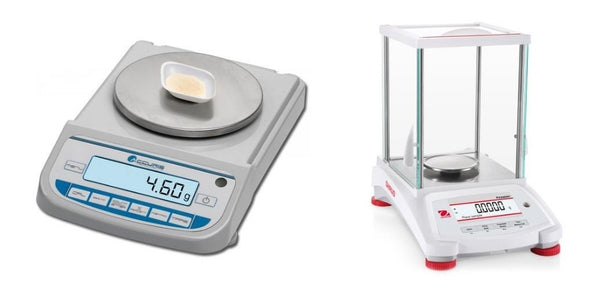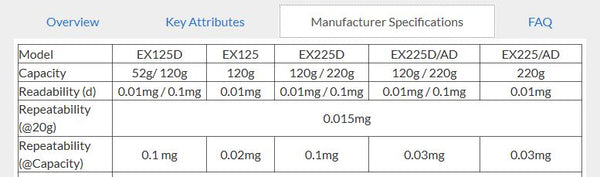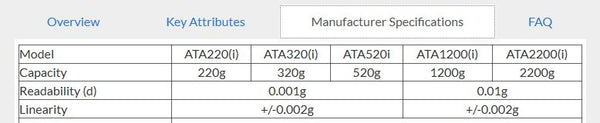# Determining if a Lab Balance Is Accurate Enough

By Aimee O'Driscoll, 17 April 2019

When you use a lab balance, how accurate you want your reading to be will depend on your application. So how do you know if the balance you’re using is going to give you the level of accuracy you need? The readability is one component but doesn’t tell the whole story.

In this post, we discuss readability and accuracy, and reveal how to determine if a balance is suitable for your application.

Readability refers to the lowest digit or quantity by which you can read a weight on a given balance. For example, many precision balances will allow you to read to 1mg, whereas many analytical balances will give readings to 0.1mg.Accuris™ Precision Balances have a readability of 1mg or 10mg (depending on the model) and Pioneer® Analytical Balances have a readability of 0.1mg.

Generally, the lower the readability, the more accurate the balance will be. That being said, readability is not the same thing as accuracy. Accuracy refers to how closely the determined result matches the true value.

## Repeatability and Maximum Uncertainty Tolerance

United States Pharmacopeia (USP) outlines a simple method to determine the minimum weight for which a balance will be accurate (the formula is provided in the next section). This takes into account a couple of other parameters: repeatability and maximum uncertainty tolerance.

• Repeatability: This tells you the ability of the balance to repeat the same result for a given load under the same conditions. The repeatability value is often the same as the readability, but may be different in some cases. Repeatability can change depending on the load, so may be provided for different masses in the manufacturer specifications. This value is calculated as a standard deviation of multiple tests (usually 10 or more), and is denoted as Rstd.Manufacturer specifications for Explorer® Semi-Micro Balances with repeatability shown for a 20g load and at capacity for each model.

• Maximum uncertainty tolerance: This defines your acceptable level of uncertainty. The higher the accuracy you need, the lower your tolerance will be for uncertainty. For example, a maximum uncertainty tolerance of 1% means you require less accuracy than if your maximum uncertainty tolerance in 0.1%.

While there is a thorough method for estimating uncertainty for a given balance, for many applications, the quick alternative method described below will suffice.

## Calculating the Minimum Weight You Can Use Your Balance For

Dividing the standard deviation of the repeatability of the balance by the maximum uncertainty tolerance will give you an estimate of the minimum weight for which a balance will be accurate enough for your application. USP uses a safety factor of two, giving the following equation:

W = 2 x Rstd / T

Where:

• W = minimum weight for which the balance will deliver an accurate enough result
• R = repeatability
• T = maximum uncertainty tolerance

Take this example:

You want to weigh a 100mg sample and need to decide if a balance will give you an accurate enough reading. The repeatability of your balance is 0.01mg and your maximum uncertainty tolerance is 0.1%. Plugging those into the equation:

W = 2 x 0.01 / 0.001 = 20mg

Since the balance will be accurate enough to weigh 20mg, you know you’ll be fine to weigh 100mg. However, if you want to weigh a 10mg sample with the same maximum uncertainty tolerance, a balance with 0.01mg repeatability won’t be suitable.

Note that you also need to consider the maximum load tolerance of a balance when deciding which to use.

## Linearity and Eccentricity

Other indicators of the accuracy of a balance are linearity and eccentricity and you may have permissible values for each of these.

Linearity tests use two weights to test the ability of the balance to measure the weight of a load before and after a second load has been placed on the pan.

The goal of an eccentricity test is to determine the ability of the balance to accurately weigh loads placed in different positions on the load receptor. This ensures that the same reading is generated no matter where the load is placed.

Manufacturer specifications often list linearity, and you should be able to obtain both linearity and eccentricity results from the manufacturer directly if not already listed.Manufacturer specifications for ATA Compact Balances showing linearity for various models.